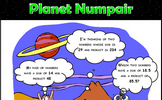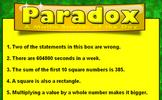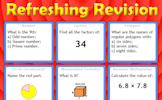# Rates

### Term 3 starting in week 5 :: Estimated time: 2 weeks

• Solve speed, distance and time problems without a calculator
• Solve speed, distance and time problems with a calculator
• Use distance/time graphs
• Solve problems with density, mass and volume
• Solve flow problems and their graphs
• Rates of change and their units

For higher-attaining pupils:

• Convert compound units

This page should remember your ticks from one visit to the next for a period of time. It does this by using Local Storage so the information is saved only on the computer you are working on right now.

## Lesson Starters

Here are some suggestions for whole-class, projectable resources which can be used at the beginnings of each lesson in this block.

### 1st Lesson#### Fly Catching

Find which whole number divided by another whole number gives the answer: 1.090909091

### 2nd Lesson#### How many of Each?

Pens cost 12p each, Pencils cost 9p each, Richard spent 72p altogether. How many pens and pencils did he buy?

### 3rd Lesson#### Nine Digit Sum

Arrange the digits one to nine to make a correct addition calculation.

### 4th LessonCan you solve this paradox by agreeing whether the statements are right or wrong?

### 5th Lesson#### Four's Independence

A clock face containing only the number 4. Can you make a clock face containing any other single number?

### 6th Lesson#### Refreshing Revision

It is called Refreshing Revision because every time you refresh the page you get different revision questions.

Some of the Starters above are to reinforce concepts learnt, others are to introduce new ideas while others are on unrelated topics designed for retrieval practice or and opportunity to develop problem-solving skills.

White Rose ResourcesEnd of block assessments provide a quick progress check at the end of each block of learning to make sure students have understood the content covered. This Scheme of Learning was produced by White Rose Maths and is used here with permission granted on 30th June 2021.For All: